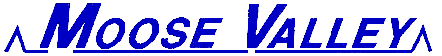Useful formulas for calculating scale measurements.

HO Scale is 1/87

To determine the Scale distance of an object when the real length in inches is known:

(Real Inches X 87) divided by 12 = HO Scale feet.

To determine the real distance in Inches when the Scale distance is known in feet:

(Scale distance X 12) divided by 87 = Real Inches